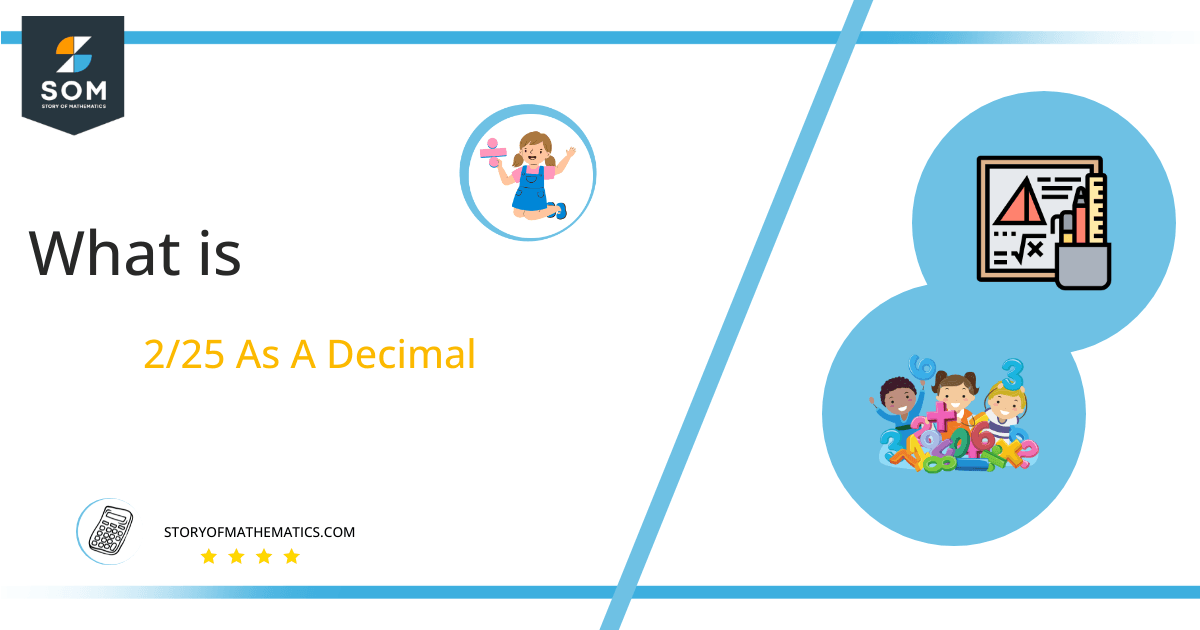# What Is 2/25 as a Decimal + Solution With Free StepsThe fraction 2/25 as a decimal is equal to 0.08.

Fraction is a mathematical concept that allows us to figure out the number of equal parts into which we can divide an object or quantity. In its representation, its two components are separated from one another by a line. The component on top of the dash or line is regarded as the Numerator, and the component at the bottom is recognized as the Denominator. While the Division process is depicted by the line between these two.

In most cases, it is desirable to change fractions into their decimal representations because the decimals are simpler to work with and comprehend than fractions. There are several ways to carry out this conversion. Long Division is one of them.

This article provides a detailed explanation of converting a fraction of 2/25 into its decimal value.

## Solution

A fraction must initially be changed into a division to resolve. We accomplish this by classifying its elements of fraction, depending on their function. As a consequence, we get the fraction’s numerator as a number, which we have to divide. And denominator is the number that will divide it.

The given example, therefore, yields a dividend of 2 and a divisor of 25.

Dividend = 2

Divisor = 25

The result obtained by the division of two numbers is known as Quotient. The term Remainder is used when there is a value that is still present after the division.

Quotient = Dividend $\div$ Divisor = 2 $\div$ 25Figure 1

## 2/25 Long Division Method

We are trying to solve the fraction 2/25.

2 $\div$ 25

To begin resolving a division issue, the type of fraction must be determined. If the fraction we were given was proper, it would have a smaller dividend than the divisor and require a decimal point in the quotient. Whereas when the fraction is incorrect, it does not require a Decimal Point because its equivalent value is higher than 1.

As it can be seen that the presented fraction of 2/25 has a denominator or divisor that is more than the dividend. Because of this, we will add a decimal point to the quotient, which is accomplished when the dividend is multiplied by 10. Multiplying the dividend 2 by 10 results in the value of 20, which is then divided by 25.

20 $\div$ 25 = 0

25 x 0 = 0

20 – 0 = 20 is the left-over value. The remainder is once more multiplied by 10, but this time decimal point is not required to be inserted in the quotient again. We now have 200 to divide by 25.

200 $\div$ 25 = 0

25 x 8 = 200

Nothing remains behind, at this point.

200-200 = 0

Zero remainder indicates that further division is not required, and the fraction is thus transformed to its appropriate decimal value, which is 0.08.Images/mathematical drawings are created with GeoGebra.